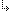- 2.2.6.13. -2. TMT Pascal Language Description2.2. Pascal Language Structure2.2.6. Types2.2.6.13. Record Types

## 2.2.6.13. Record Types

Record types are structures that contain components of different types. Each component of a record is called a field. Variant sections are parts of records can have multiple definitions. Record type are defined as follows:
```  record
Fieldlist
end```
where Fieldlist is defined as:
`  [[Fixedpart] | [Fixedpart;] [Variantpart]];`
where Fixedpart is:
`  Field [; Field];`
where Field is
`  identifier [,identifier] : Identifiertype;`
Variantpart is defined as follows:
```case [identifier:] Typename of
Variant
[;Variant];```
where Variant is:
`  Caserange [,Caserange] : (Fieldlist);`
where Caserange is:
`  expression [..expression];`
With a proper understanding of TMT Pascal record types, very powerful types can be defined. The following are examples of record types:
```type
Coordinate = record
x, y: Integer;
end;
Values = record
case Way: Boolean of
True:  (RValue : Extended);
False: (IValue : Longint);
end;```
To reference a field of a record specify the record variable followed by a period (.) followed by the field name. The following refers to the fields of Coordinate declared above:
```  Coordinate.x
Coordinate.y```

- 2.2.6.13. -